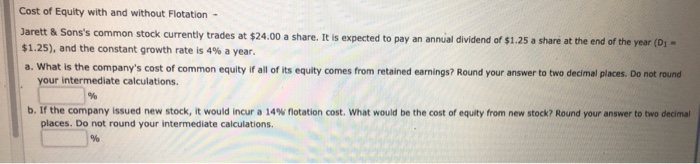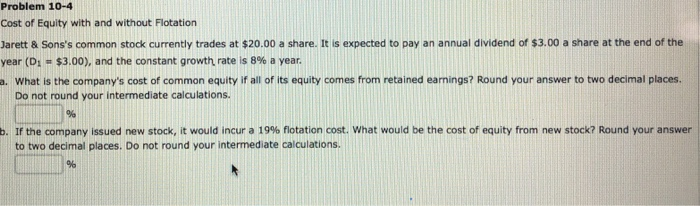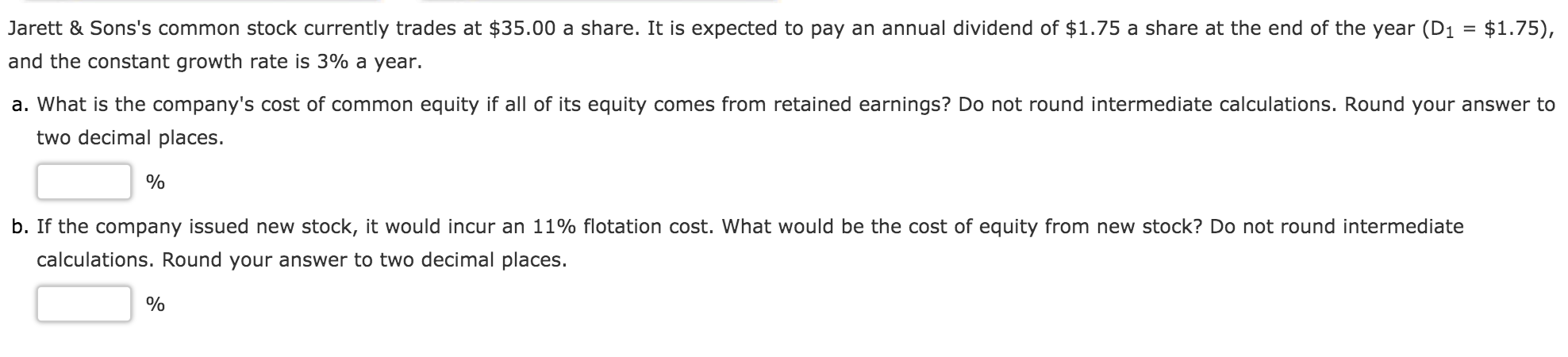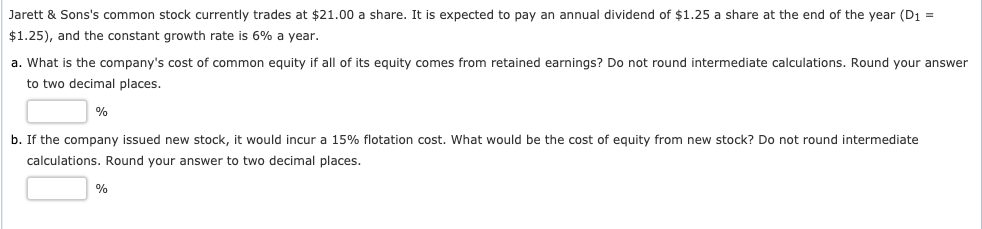Question

# COST OF EQUITY WITH AND WITHOUT FLOTATION Jarett & Sons's common stock currently trades at \$39.00...

COST OF EQUITY WITH AND WITHOUT FLOTATION

Jarett & Sons's common stock currently trades at \$39.00 a share. It is expected to pay an annual dividend of \$3.00 a share at the end of the year (D1 = \$3.00), and the constant growth rate is 5% a year.

1. What is the company's cost of common equity if all of its equity comes from retained earnings? Round your answer to two decimal places. Do not round your intermediate calculations.
%

2. If the company issued new stock, it would incur a 8% flotation cost. What would be the cost of equity from new stock? Round your answer to two decimal places. Do not round your intermediate calculations.
%

a.Cost of equity=(D1/Current price)+Growth rate

=(3/39)+0.05

=12.69%(Approx).

b.Cost of equity=(D1/Current price(1-Flotation cost)+Growth rate

=(3/39(1-0.08))+0.05

=(3/35.88)+0.05

=13.36%(Approx).

#### Earn Coins

Coins can be redeemed for fabulous gifts.

Similar Homework Help Questions
• ### Cost of Equity with and without Flotation Jarett & Sons's common stock currently trades at \$26.00...

Cost of Equity with and without Flotation Jarett & Sons's common stock currently trades at \$26.00 a share. It is expected to pay an annual dividend of \$1.75 a share at the end of the year (D1 = \$1.75), and the constant growth rate is 5% a year. What is the company's cost of common equity if all of its equity comes from retained earnings? Round your answer to two decimal places. Do not round your intermediate calculations. % If...

• ### Cost of Equity with and without Flotation Jarett & Sons's common stock currently trades at \$22.00...

Cost of Equity with and without Flotation Jarett & Sons's common stock currently trades at \$22.00 a share. It is expected to pay an annual dividend of \$2.75 a share at the end of the year (D1 = \$2.75), and the constant growth rate is 7% a year. What is the company's cost of common equity if all of its equity comes from retained earnings? Round your answer to two decimal places. Do not round your intermediate calculations. % If...

• ### Cost of Equity with and without Flotation - Jarett & Sons's common stock currently trades at...Cost of Equity with and without Flotation - Jarett & Sons's common stock currently trades at \$24.00 a share. It is expected to pay an annual dividend of \$1.25 a share at the end of the year (D \$1.25), and the constant growth rate is 4% a year. a. What is the company's cost of common equity If all of its equity comes from retained earnings? Round your answer to two decimal places. Do not round your intermediate calculations b....

• ### Problem 10-4 Cost of Equity with and without Flotation Jarett & Sons's common stock currently trades...Problem 10-4 Cost of Equity with and without Flotation Jarett & Sons's common stock currently trades at \$20.00 a share. It is expected to pay an annual dividend of \$3.00 a share at the end of the year (D: - \$3.00), and the constant growth rate is 8% a year. What is the company's cost of common equity if all of its equity comes from retained earnings? Round your answer to two decimal places. Do not round your intermediate calculations....

• ### Jarett & Sons's common stock currently trades at \$25.00 a share. It is expected to pay...

Jarett & Sons's common stock currently trades at \$25.00 a share. It is expected to pay an annual dividend of \$3.00 a share at the end of the year (D1 = \$3.00), and the constant growth rate is 4% a year. What is the company's cost of common equity if all of its equity comes from retained earnings? Do not round intermediate calculations. Round your answer to two decimal places.   % ? If the company issued new stock, it would...

• ### Jarett & Sons's common stock currently trades at \$40.00 a share. It is expected to pay...

Jarett & Sons's common stock currently trades at \$40.00 a share. It is expected to pay an annual dividend of \$1.50 a share at the end of the year (D1 = \$1.50), and the constant growth rate is 7% a year. What is the company's cost of common equity if all of its equity comes from retained earnings? Do not round intermediate calculations. Round your answer to two decimal places. If the company issued new stock, it would incur a...

• ### Jarett & Sons's common stock currently trades at \$37.00 a share. It is expected to pay...

Jarett & Sons's common stock currently trades at \$37.00 a share. It is expected to pay an annual dividend of \$2.25 a share at the end of the year (D1 = \$2.25), and the constant growth rate is 4% a year. What is the company's cost of common equity if all of its equity comes from retained earnings? Round your answer to two decimal places. Do not round your intermediate calculations. % If the company issued new stock, it would...

• ### Jarett & Sons's common stock currently trades at \$29.00 a share. It is expected to pay...

Jarett & Sons's common stock currently trades at \$29.00 a share. It is expected to pay an annual dividend of \$1.50 a share at the end of the year (D1 = \$1.50), and the constant growth rate is 3% a year. a. What is the company's cost of common equity if all of its equity comes from retained earnings? Round your answer to two decimal places. Do not round your intermediate calculations. b. If the company issued new stock, it...

• ### Jarett & Sons's common stock currently trades at \$35.00 a share. It is expected to pay...Jarett & Sons's common stock currently trades at \$35.00 a share. It is expected to pay an annual dividend of \$1.75 a share at the end of the year (D1 = \$1.75), and the constant growth rate is 3% a year. a. What is the company's cost of common equity if all of its equity comes from retained earnings? Do not round intermediate calculations. Round your answer to two decimal places. % b. If the company issued new stock, it...

• ### Jarett & Sons's common stock currently trades at \$21.00 a share. It is expected to pay...Jarett & Sons's common stock currently trades at \$21.00 a share. It is expected to pay an annual dividend of \$1.25 a share at the end of the year (D1 = \$1.25), and the constant growth rate is 6% a year. a. What is the company's cost of common equity if all of its equity comes from retained earnings? Do not round intermediate calculations. Round your answer to two decimal places. b. If the company issued new stock, it would...